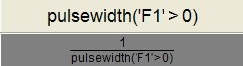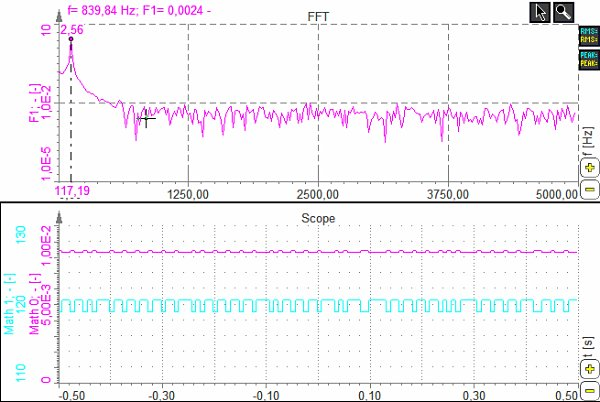# Example of measure functions

The following example shows the measurement of the pulsewidth. Let’s say that the input is a square wave and we want to measure the pulse width of this channel. First of all, we need to translate the input signal in the logic with ‘F1’>0. This will give a value of 1 for positive and value of zero for negative values of the input signal. The pulsewidth function will then measure the time between each transition. If we want to measure the frequency, we can calculate the inverse of this function as seen below.The graph shows a pulse width measurement (Math0) and frequency measurement (Math1).‘pulsewidth’ function is useful for digital signal. The resolution of the measurement is limited by the sample rate.

If we want to measure the pulse width or frequency of sine waves, it is better to use Exact frequency math module, described in the following chapters.